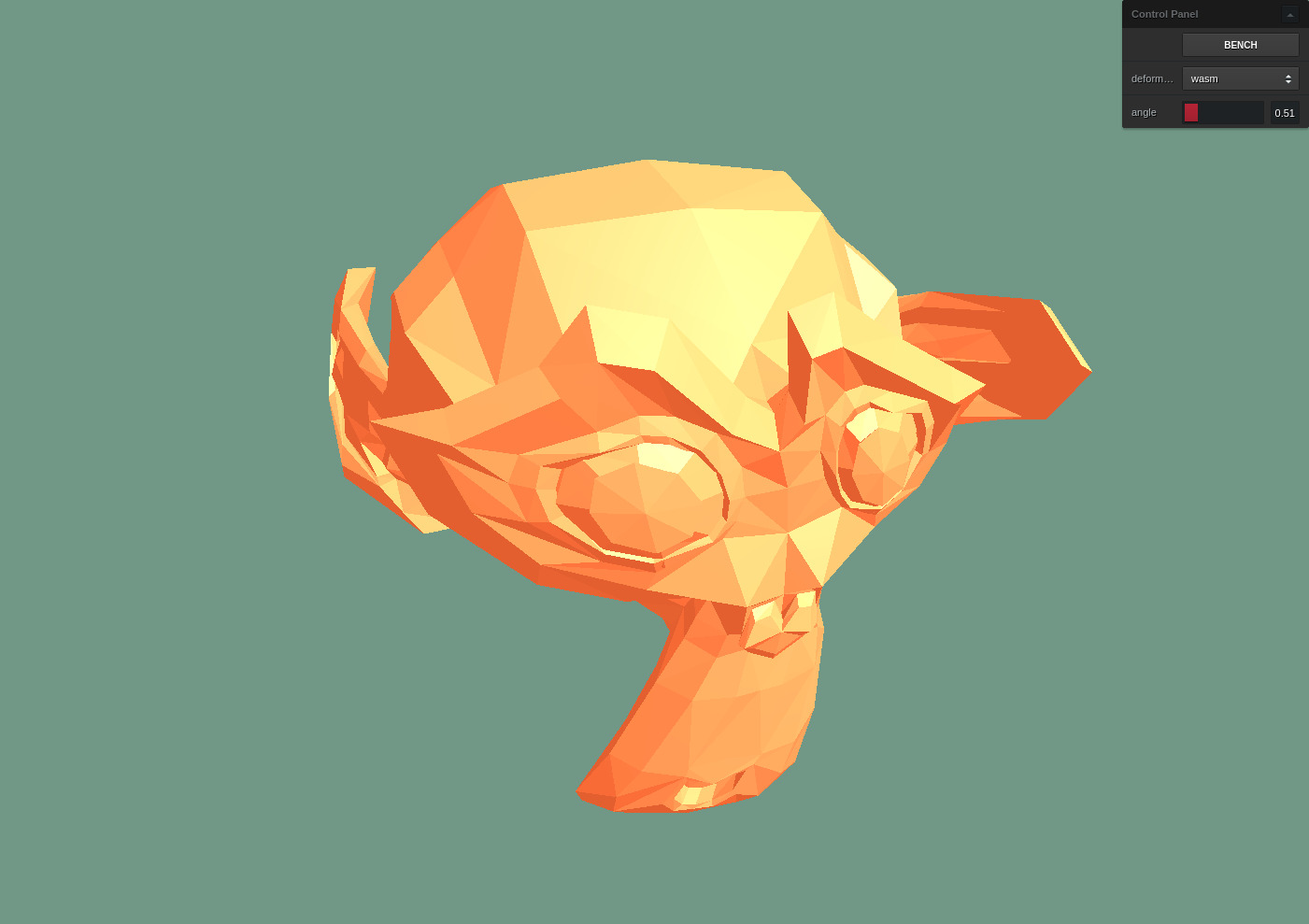# Deforming a THREE.js mesh with Rust

#### A little journey into WebAssembly

A while ago I worked on a project which allowed users to deform a 3D mesh in interesting ways. A vertex shader would be the usual weapon for something like this, but in this case we had to store the resulting deformed mesh, so it was simpler to implement in Javascript.

I wondered at the time how much faster Rust and WebAssembly could be, and I've finally got around to giving it a go...I've chosen one simple deformation to make it easy to compare - it's essentially rotating vertices in proportion to their distance from the origin, which gives a twisting effect. Here's the Javascript implementation:

``````function deformer(position, origPosition, angle) {
for (
let i = 0, last = position.length;
i < last;
i += 3
) {
const x = origPosition[i],
y = origPosition[i + 1],
z = origPosition[i + 2];

const lenSq = (x * x) + (y * y) + (z * z);
const theta = lenSq * angle;
const c = Math.cos(theta);
const s = Math.sin(theta);

position[i] = c * x - s * z;
position[i + 1] = y;
position[i + 2] = s * x + c * z;
}
}``````

The Rust version is naturally very similar with a bit of wasm-bindgen boilerplate:

``````use wasm_bindgen::prelude::*;

#[wasm_bindgen]
pub fn deformer(
position: &mut [f32],
orig_position: &[f32],
angle: f32,
) {
for i in (0..position.len()).step_by(3) {
let x = orig_position[i];
let y = orig_position[i + 1];
let z = orig_position[i + 2];

let len_sq = (x * x) + (y * y) + (z * z);
let theta = len_sq * angle;
let c = theta.cos();
let s = theta.sin();

position[i] = c * x - s * z;
position[i + 1] = y;
position[i + 2] = s * x + c * z;
}
}``````

Using Parcel for the bundler, integrating the Rust version is ridiculously simple. You can literally import the Rust package in Javascript:

``import deformer from '../deformer-rs/Cargo.toml';``

One quick note, I first tried with an alpha version of Parcel 2 but it seems WebAssembly support is still in progress, so I ended up with Parcel 1.x and parcel-plugin-wasm.rs.

### Performance

Although I haven't tested this in the most scientific manner (a quick and dirty benchmark script on a single laptop!) I'm surprised by the results.

I've seen very little difference in performance in Chrome. Averaging over 20 runs the WebAssembly version is about 10% faster.

There's a larger difference in Firefox with the WebAssembly version around 40% faster than the Javascript version, but this is more of a credit to Chrome's Javascript engine than a shining example of WebAssembly:

Browser Javascript WebAssembly
Chromium 79.0.3945.130 (Linux 64-bit) 51ms 44ms
Firefox 68.4.1esr (Linux 64-bit) 70ms 40ms

As you'd expect a lot of the running time is due to the two trigonometric function calls per vertex. Replacing these with simple multiplications makes everything faster but doesn't change the rankings - WebAssembly is still a bit faster and Chrome's Javascript runtime is still very close behind.

Finally it's worth noting that for a task like this where every vertex is calculated independently you could use multiple Web Workers. Theoretically even using two Javascript workers would provide more of a speedup.

You can browse the whole project and make fun of my typos fork it on GitHub.